Mathematical Logic as based on the Theory of Types

BY   BERTRAND  RUSSELL

Published in: American Journal of Mathematics, vol.30(1908), pp. 222-262
Transcribed into hypertext by Burtzev B.I., Jul., 07, 2003
e-mail: bbi-math@narod.ru,   site: http://bbi-math.narod.ru/

VII.

Elementary Theory of Classes and Relations.

EG.Propositions in which a function φ occurs may depend, for their truth-value, upon the particular function φ, or they may depend only upon the extension of φ, i.e., upon the arguments which satisfy φ. A function of the latter sort we will call extensional. Thus, e.g., УI believe that all men are mortalФ may not be equivalent to УI believe that all featherless bipeds are mortal,Ф even if men are coextensive with featherless bipeds; for I may not know that they are coextensive. But Уall men are mortalФ must be equivalent to Уall featherless bipeds are mortalФ if men are coextensive with featherless bipeds. Thus Уall men are mortalФ is an extensional function of the function Уx is a man,Ф while УI believe all men are mortalФ is a function which is not extensional; we will call functions intensional when they are not extensional. The functions of functions with which mathematics is specially concerned are all extensional. The mark of an extensional function f of a function φ!z^   is

φ!x.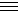x . ψ!x: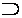φ, ψ : f (φ!z^).. f(ψ!z^)
EH.From any function f of a function φ!z^   we can derive an associated exten-sional function as follows. Put

f { z^ (ψz)} . = : (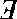φ):φ!x.x . ψx:f{φ ! z^}  Df.

The function f { z^ (ψz)} is in reality a function of ψz^ though not the same function as f ( ψz^ ), supposing this latter to be significant. But it is convenient to treat f { z^ ( ψz ) } technically as though it had an argument  z^ ( ψz ) , which we call Уthe class defined by ψФ We have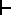:. φx.x .ψx::f { z^ (φz)} .. f { z^ (ψz)},

whence, applying to the fictitious objects z^ (φz) and z^ (ψz) the definition of identity given above, we find:. φx.x .ψx:. z^ (φz) = z^ (ψz).

EI.This, with its converse (which can also be proved), is the distinctive property of classes. Hence we are justified in treating z^ (φz) as the class defined by φ. In the same way we put

f { x^ y^ ψ(x, y)} . = : (φ):φ!(x, y).x, y . ψ( x, y):f{φ ! ( x^ y^)}  Df.

A few words are necessary here as to the distinction between φ ! ( x^ y^) and φ ! ( y^ x^). We will adopt the following convention: When a function (as opposed to its values) is represented in a form involving x^ and y^, or any other two letters of the alphabet, the value of this function for the arguments a and b is to be found by substituting a for x^ and b for y^; i.e., the argument mentioned first is to be substituted for the letter which comes earlier in the alphabet, and the argument mentioned second for the later letter. This sufficiently distinguishes between φ ! ( x^ y^) and φ ! ( y^ x^); e.g.:
The value of φ ! ( x^ y^) for arguments a, b is φ!(a, b).
The value of φ ! ( x^ y^) for arguments b, a is φ!(b, a).
The value of φ ! ( y^ x^) for arguments a, b is φ!(b, a).
The value of φ ! ( y^ x^) for arguments b, a is φ!(a, b).
We put
x ε φ! z^ . = . φ!x  Df.

whence:. x εz^ (ψz). = :(φ) : φ ! y.y . ψy : φ ! x.

Also by the reducibility-axiom we have

(φ) : φ ! y.y . ψy,

whence: x εz^ (ψz).. ψx.

EJ.This holds whatever x may be. Suppose now we want to consider z^(ψz) ε φ^ f {z^ ! z)}.We have, by the above,:. z^ ( ψz ) ε φ^ f {z^ ! z)}.: f {z^(φz)} ::(φ):φ ! y .y . ψy : f {φ ! z},

whence:. z^ ( ψz) = z^ (χz ) .: z^ (ψz ) ε κ .κ . z^ (χz) ε κ,

where κ is written for any expression of the form φ^ f {z^ ! z)}.
EK.We put
cls = α^ {(φ ) . α = z^ ( φ ! z) }   Df.

Here cls has a meaning which depends upon the type of the apparent variable φ. Thus, e.g., the proposition Уcls ε clsФ which is a consequence of the above definition, requires that УclsФ should have a different meaning in the two places where it occurs. The symbol УclsФ can only be used where it is unnecessary to know the type; it has an ambiguity which adjusts itself to circumstances. If we introduce as an indefinable the function УIndiv!xФ meaning Уx is an individual,Ф we may put

Kl = α^ {(φ ) . α = z^ ( φ ! z . Indiv ! z) }   Df.

Then Kl is an unambiguous symbol meaning Уclasses of individuals.Ф
EL.We will use small Greek letters (other than ε, φ, ψ, χ, θ ) to represent classes of whatever type; i.e., to stand for symbols of the form z^ ( φ ! z ) or z^ ( φz ).
EM.The theory of classes proceeds, from this point on, much as in Peano's system; z^ (φz ) replaces   z ε (φz ). Also I put
 α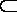β . = :x ε α .x . x ε β Df.! α . = . (x) . x ε α Df. V = x^ ( x = x) Df. Λ = x{~ ( x = x)} Df.
where Λ, as with Peano, is the null-class. The symbols, Λ, V, like cls and ε, are ambiguous, and only acquire a definite meaning when the type concerned is otherwise indicated.
EN.We treat relations in exactly the same way, putting

α{φ ! ( x^ , y^}b . = . φ ! ( a, b)    Df.

(the order being determined by the alphabetical order of x and ,y and the typographical order of a and b); whence:. a { x^ y^ψ( x, y) } b .: (φ) : ψ( x, y) .x, y . φ ! ( x, y) : φ ! (a, b),

whence, by the reducibility-axiom,: a { x^ y^ψ( x, y) } b .. ψ(a, b).

EO.We use Latin capital letters as abbreviations for such symbols as x^ y^ψ( x, y), and we find:. R = S .: xRy .x, y . xSy,

where

R = S . = :f !R .f .  f ! S   Df.

We put

Rel = R^ {(φ) . R = x^ y^ φ ! ( x, y) }   Df.

and we find that everything proved for classes has its analogue for dual relations. Following Peano, we put

α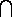β = x^ (x ε α . x ε β)   Df.,

defining the product, or common part, of two classes;

α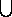β = x^ (x ε α .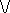. x ε β)   Df.,

defining the sum of two classes; and

- α = x^{ ~( x ε α) }   Df.,

defining the negation of a class. Similarly for relations we put
 R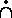S = x^ y^ (xRy . xSy) Df. R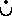S = x^ y^ (xRy .. xSy) Df.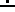R = x^ y^ {~ (xRy)} Df.

 Contents: I.    The Contradictions. II.   All and Any III.  The Meaning and Range of Generalized Propositions. IV.   The Hierarchy of Types. V.    The Axiom of Reducibility. VI.   Primitive Ideas and Propositions of Symbolic Logic. VII.  Elementary Theory of Classes and Relations. VIII. Descriptive Functions. IX.   Cardinal Numbers. X.    Ordinal Numbers. —одержание: I.    ѕарадоксы. II.   “¬се, каждый”   и   “любой, произвольный” III. —мысл и область применимости обобще... IV. V. VI. VII. VIII. IX. X.